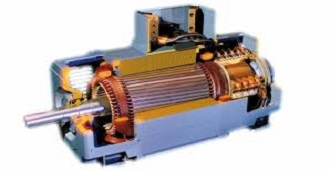# AC MotorsEectrical machines & drive control course

Part 1: Electrical machines

Introduction and Concepts of magnetic circuits

1- Three phase induction motor

• Construction and design
• Equivalent circuit and parameters calculation and Tests
• Types of 3ph IM (slip rings – squirrel cag – deep bar- double cage )
• The motor characteristics for Power & torque _ speed& current _ speed
• Starting methods
• Speed control and Torque control
• Winding rules
• Electrical power recovery
• Electrical braking of induction motor
• 3 phase motor converted to single phase
• power factor correction

2- Single phase Induction motors

• Theory of work and Circuit analysis
• Equivalent circuit
• Capacitor run motor
• Capacitor start motor
• Capacitor start Capacitor run motor
• Split phase motor
• 3ph winding for single phase motor
• Starting and speed control and Torque control
• Winding rules

3- Universal motor

Series universal motor

• Construction and Applications
• Examples
• Speed control and Torque control

Differential universal motor

• Theory of work
• Construction and Applications

Schrage motor

• Theory of work
• Construction and Applications

4- Three phase induction Generator

• Theory of work
• Equivalent circuit and parameters calculation
• Types of excitation for induction Generator

5- DC generators

• Theory of working
• Construction
• Armature reaction and how it affects
• Types of dc generators and methods of excitation
• Electrical external curves
• voltage regulation
• Calculations and examples
• Applications

6- DC motors

• Theory of working
• Construction
• Electro motive force & torque equation
• Types of dc motors
• Speed and torque curves
• Calculations and Applications
• Starting & reverse direction
• Speed control of dc motors
• Electrical braking of dc motors

7- Synchronous Generators

• Theory & construction
• Equivalent circuit
• Vector diagram and Tests
• Voltage regulation and Power & torque
• Parallel operation

8-Synchronous Motors

• Theory & construction
• Starting methods
• Power factor with synchronous motor
• Calculations of Power , torque and efficiency

9- Diesel Generator

• Theory & construction
• AVR
• Maintenance of diesel generator

10- Special machines

• Stepper motor
• Tachogenerator
• Servo motor
• induction servo motors
• Dc servo motors

Part 2 Classic Control 1-classic control component

• switches
• Relay and Contactor
• circuit breaker types
• timers (on delay timer-off-delay timer)
• limit switch
• sensors

2- Simulation of Classic control

3- Classic control application (20 applications handwork )

• Control circuits for DC motors
• Control circuits for single phase motors
• Control circuits for three phase motors
• Forward and reserve for three phase motors
• Control circuits (Delta/star ) three phase motors
• Control circuits for Dahlander motors

Part 3 Drive system

• Introduction
• Drive system components
• What is difference between inverter and a Soft Starter
• What is difference between inverter and frequency converter
• Block diagram of electric drive system
• Calculations
• Characteristic curves
• How to read the motor correctly?
• How to choose the drive type?
• Choosing type of control and protections
• Installation of the drive system
• Wiring and connections
• How to use digital inputs and analogue inputs to control the drive
• How to have a feedback from the dive and to use it in other process
• How to have a feedback from PLC
• How to collect your calculations before programming start
• Before you begin
• Steps for setting up
• Parameter setting method
• Frequency Setting via keypad & operating via terminals
• Basic Functions and Function list
• Drive Group
• Function group
• I/O configuration
• Inserting the motor data
• Calculations and insertion of protection currents
• Choosing direction of motion for forward and reverse directions
• Motion curves of acceleration and deceleration
• Motor control
• Types of stopping
• Ramp shape
• Brake release and brake engage
• Dc injection
• High speed
• more exercise for application on 3 phase induction motors